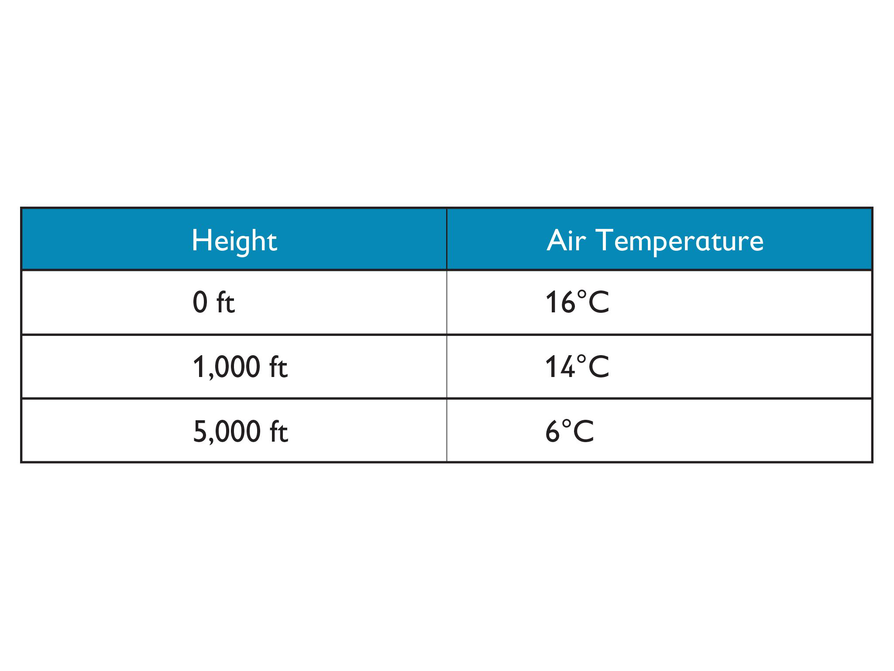Subject:
Mathematics
Material Type:
Lesson Plan
Level:
Middle School
7
Provider:
Pearson
Tags:
Language:
English
Media Formats:
Text/HTML

# Gallery Problems Exercise# Gallery Overview

Allow students who have a clear understanding of the content in the unit to work on Gallery problems of their choosing. You can then use this time to provide additional help to students who need review of the unit’s concepts or to assist students who may have fallen behind on work.

# Problem Descriptions

Multiplication Webs
Students fill in the blanks to create expressions equal to the number in the center of the web.

Number Trees
Students complete number tree puzzles.

Are They Equivalent?
Students decide when given expressions will have the same value as ab.

Squaring and Cubing
Students find solutions to simple equations and inequalities involving squares and cubes.

Transforming Triangles
Students investigate how a triangle changes when they multiply the coordinates of its vertices by positive and negative numbers.

True or False?
Students determine whether given statements about positive and negative numbers are true or false.

Saving Money
Students use positive and negative numbers to make sense of changes to Lucy’s savings account.

Altitude and Temperature
Students explore how the air temperature changes as the height of an airplane changes.

# Multiplication Webs

1. First web: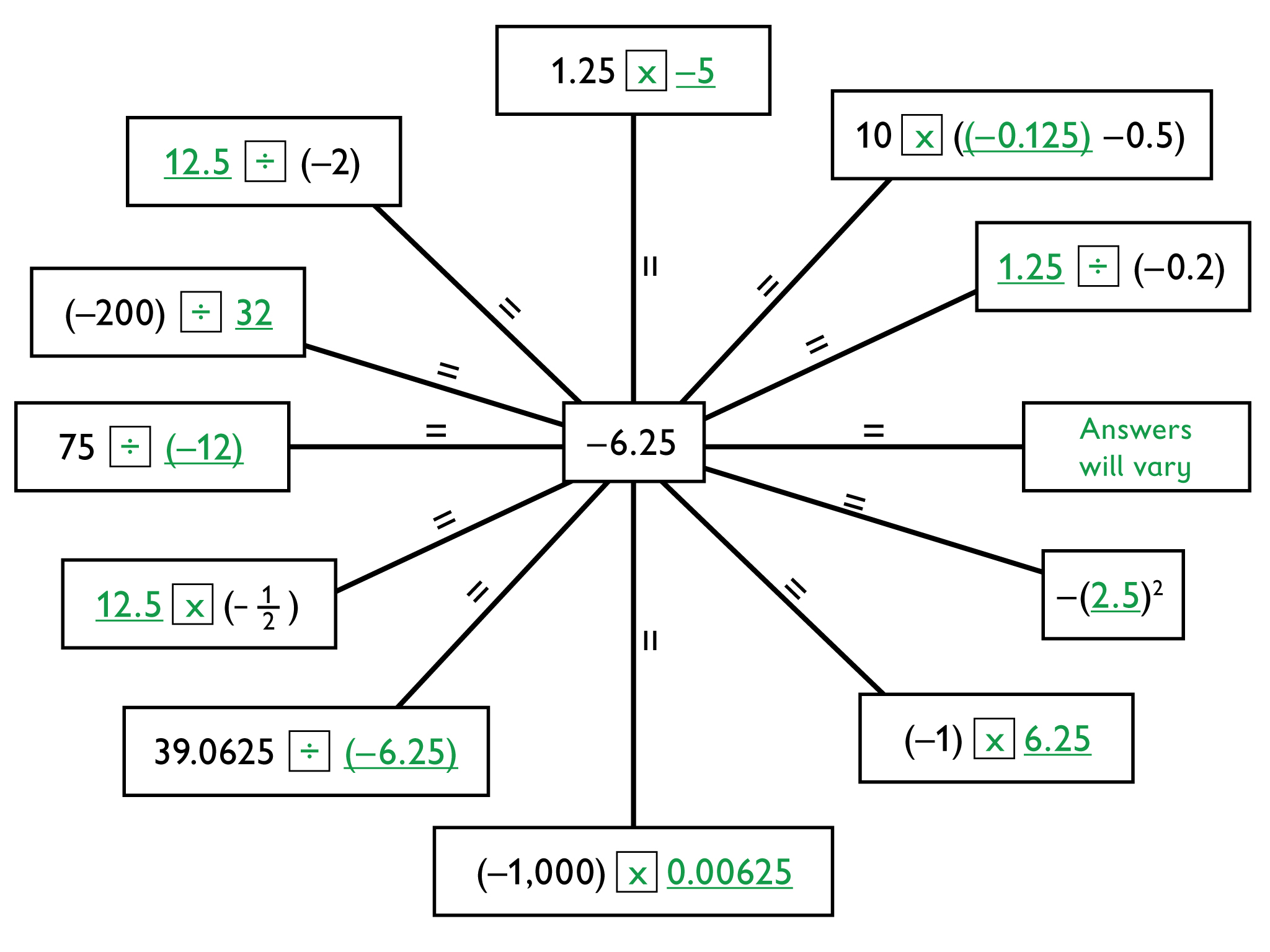2. Second web: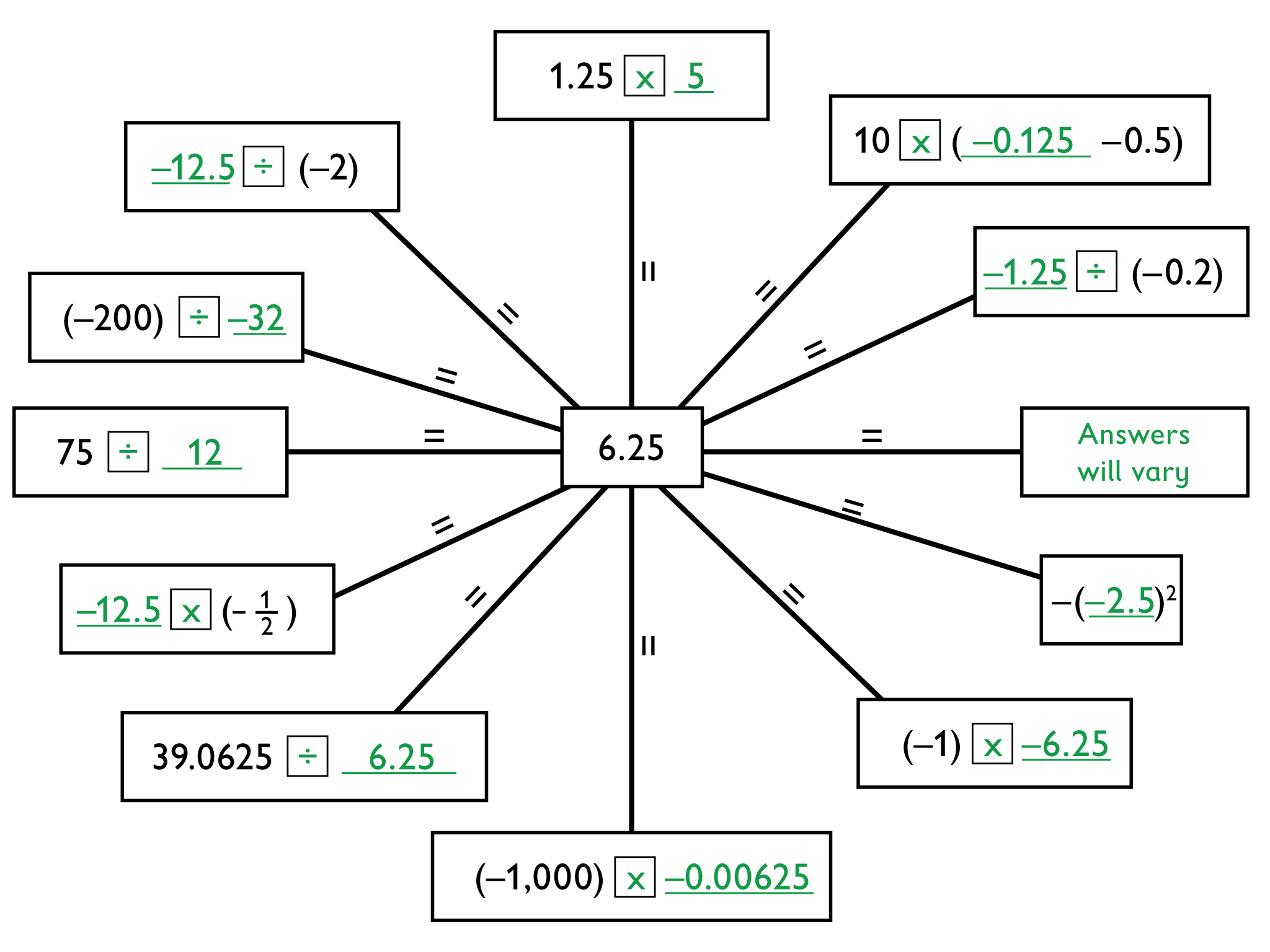# Multiplication Webs

1. Write a number on each blank line and a multiplication ($×$x) or division (÷) symbol in each box to create expressions with a value equal to the center number. In the blank rectangle, write any expression that uses multiplication or division. Make sure to complete two webs. You may use a calculator.

HANDOUT: Multiplication Web

# Number Trees

1. First tree: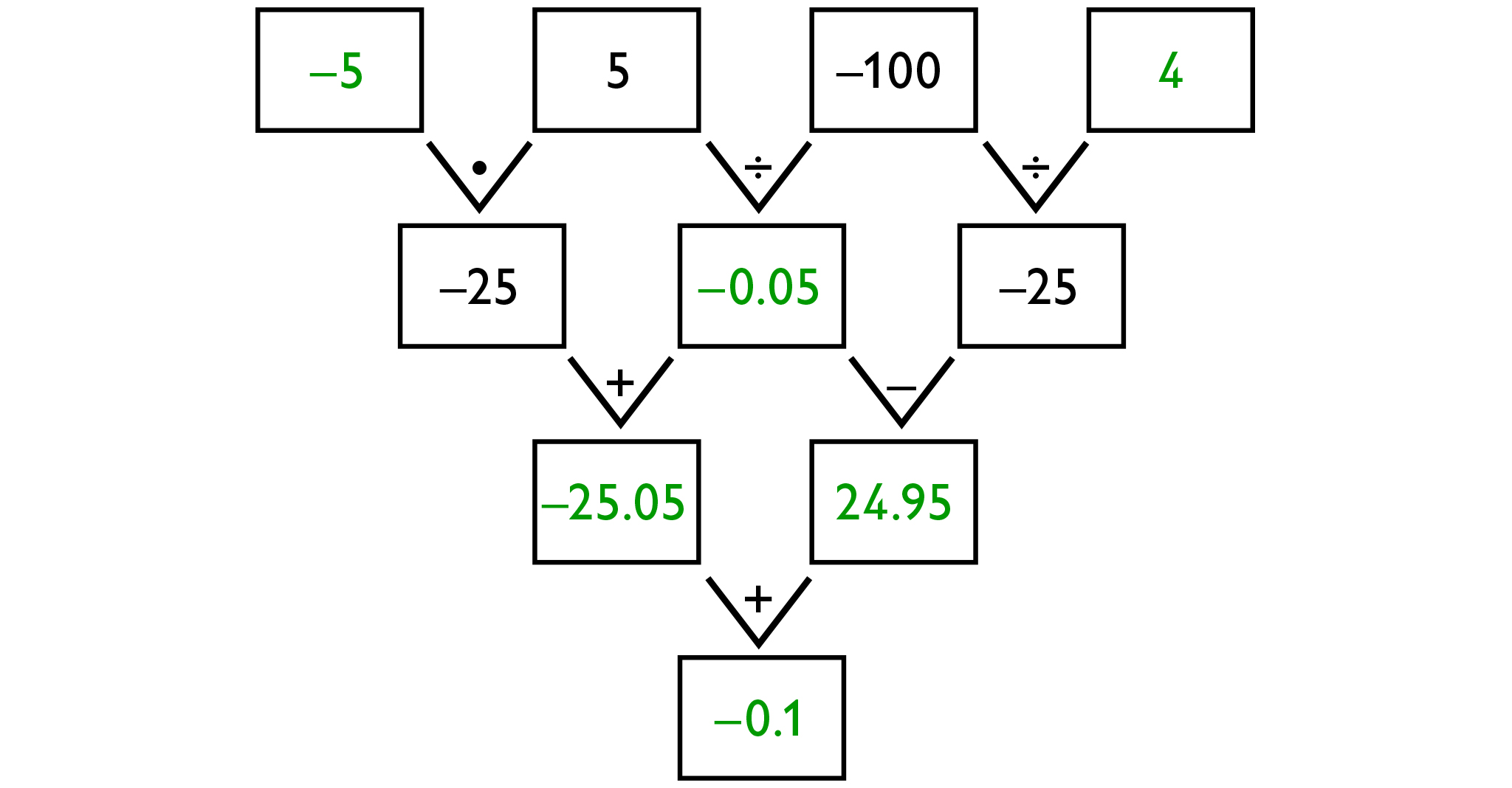2. Second tree: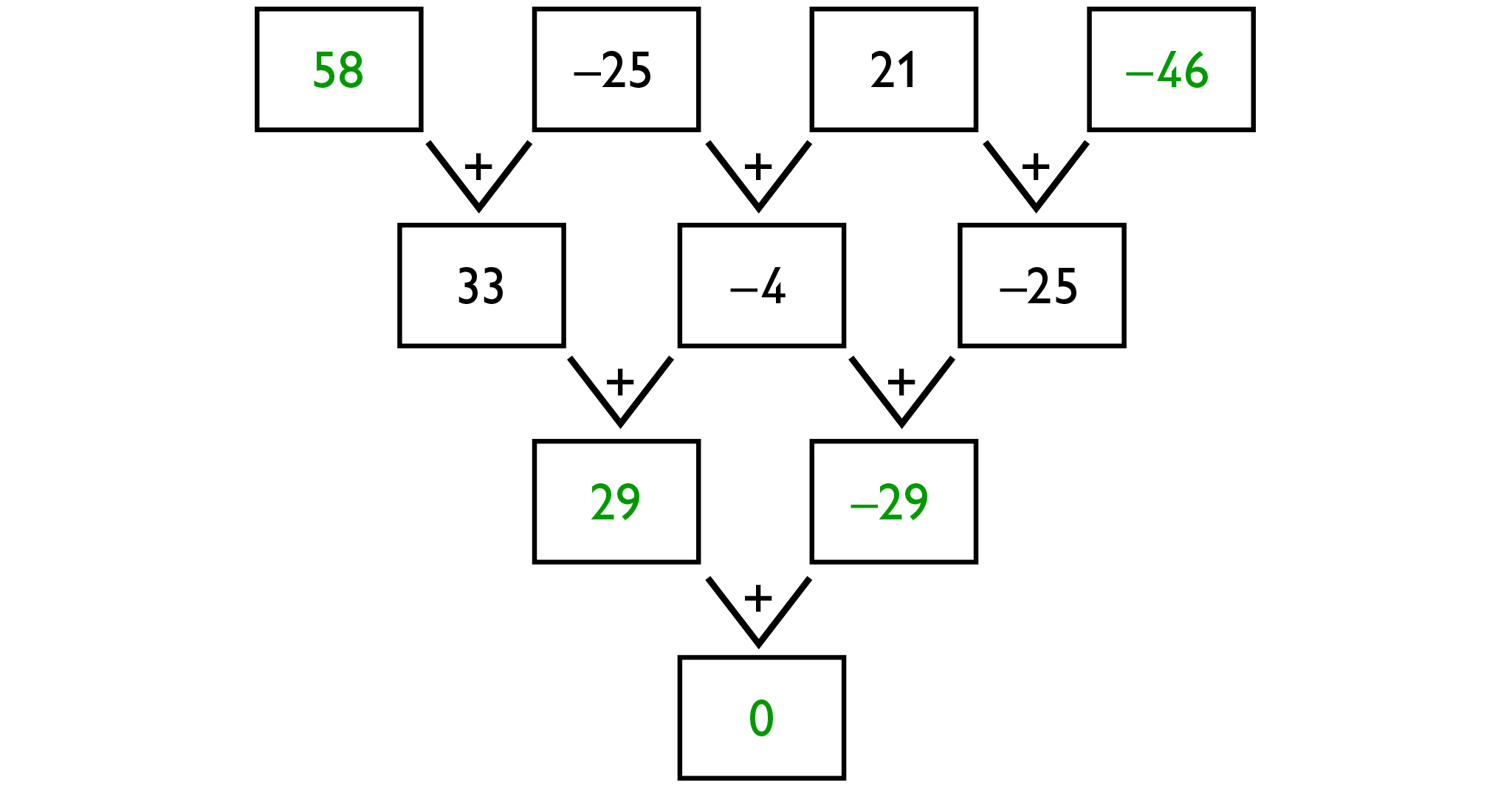# Number Trees

Complete each number tree by filling in the blanks. There are many possible answers for the third and fourth trees.

HANDOUT: Number Trees

# Are They Equivalent?

1. Expressions:

a) Equivalent: $a\cdot \frac{1}{b}=\frac{a}{1}\cdot \frac{1}{b}=\frac{a}{b}$

b) Not equivalent—for example, when a = 1 and b = −2, $\frac{a}{b}=\frac{1}{2}$, but $|\frac{a}{b}|=\frac{1}{2}$

c) Not equivalent—for example, when a = 1 and b = 2, $\frac{a}{b}=\frac{1}{2}$, but $\frac{b}{a}=2$

d) Not equivalent—for example, when a = 2 and b = 3, $\frac{a}{b}=\frac{2}{3}$ but $\frac{1}{ab}=\frac{1}{6}$

e) Equivalent: $\frac{10a}{10b}=\frac{10}{10}\cdot \frac{a}{b}=1\cdot \frac{a}{b}=\frac{a}{b}$

f) Equivalent: $\frac{-a}{-b}=\frac{-1\cdot a}{-1\cdot b}=\frac{-1}{-1}\cdot \frac{a}{b}=\frac{a}{b}$

g) Not equivalent—for example, when a = 2 and b = 3, $\frac{a}{b}=\frac{2}{3}$, but $\frac{a-1}{b-1}=\frac{1}{2}$

2. b) $\frac{a}{b}$ will have the same value as |$\frac{a}{b}$| when a and b are both positive or both negative or when a = 0.

c) $\frac{a}{b}$ will have the same value as $\frac{b}{a}$ when a = b (and neither a nor b is 0).

d) $\frac{a}{b}$ will have the same value as $\frac{1}{ab}$ when a = 1 (in which case, both expressions equal $\frac{1}{ab}$) or when a = −1 (in which case, both expressions equal −$\frac{1}{ab}$). In both cases, b must not equal 0.

g) $\frac{a}{b}$ will have the same value as $\frac{a-1}{b-1}$ when a = b and they do not equal 0 or 1, in which case, both expressions will be equal to 1.

# Are They Equivalent?

Two algebraic expressions are equivalent if they are equal for all variable values.

Decide whether each expression is equivalent to $\frac{a}{b}$. If it is, explain how you know. If it is not, give values of a and b for which the expression is not equal to $\frac{a}{b}$.

1. a) $a\cdot \frac{1}{b}$

b) $|\frac{a}{b}|$

c) $\frac{b}{a}$

d) $\frac{1}{ab}$

e) $\frac{10a}{10b}$

f) $\frac{-a}{-b}$

g) $\frac{a-1}{b-1}$

2. Consider the expressions in the previous problem that are not equivalent to $\frac{a}{b}$. Tell whether each expression would have the same value as $\frac{a}{b}$ under certain conditions. Is so, specify those conditions.

# Squaring and Cubing

1. $x=-2$ and $x=2$
2. Possible answer: $x=\frac{1}{2}$ and $x=-\frac{1}{2}$
Any number with an absolute value less than 2 is a solution.
3. Possible answer: $x=3$ and $x=-3$
Any number with an absolute value greater than 2 is a solution.
4. Possible answer: When you multiply a positive number by itself, you get a positive number. When you multiply a negative number by itself, you get a positive number. So, there is no real number you can multiply by itself to get $-9$.
5. $x=2$ is the only solution.
6. Possible answer: $x=1$ and $x=-1$
Any number less than 2 is a solution.
7. Yes. $x=\left(-2\right)$ is a solution because ${\left(-2\right)}^{3}=\left(-2\right)\cdot \left(-2\right)\cdot \left(-2\right)=-8$
8. A negative solution is $x=-3$ (or any value less than $-2$). There are no positive solutions. Any positive number cubed is positive, so ${x}^{3}$ cannot be less than $-8$ for any positive number.

# Squaring and Cubing

1. The equation ${x}^{2}=4$ has two solutions. Find them.
2. Find a positive solution and a negative solution for ${x}^{2}<4$ if possible. If it is not possible, explain why.
3. Find a positive solution and a negative solution for ${x}^{2}>4$ if possible. If it is not possible, explain why.
4. Can you find the solution for ${x}^{2}=-9$? If it is not possible, explain why it does not have a solution.
5. Find the solution(s) for ${x}^{3}=8$.
6. Find a positive solution and a negative solution for ${x}^{3}<8$ if possible. If it is not possible, explain why.
7. Does ${x}^{3}=-8$ have a solution? Explain.
8. Find a positive solution and a negative solution for ${x}^{3}<-8$ if possible. If it is not possible, explain why.

# Transforming Triangles

All the triangles for Problems 1 and 2 are shown in the graph.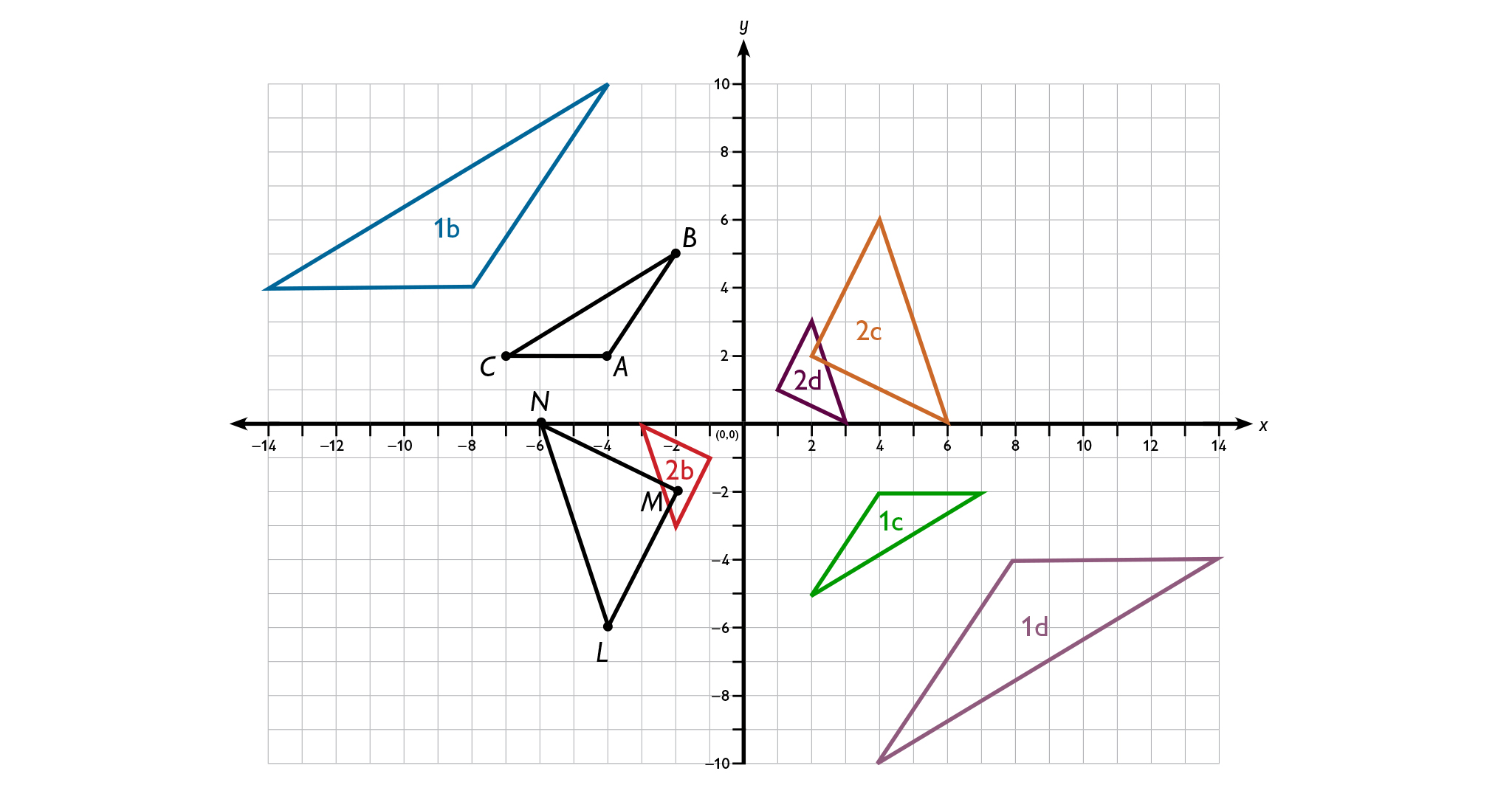1. For △ABC:

• Possible answer: The new triangle is bigger than △ABC. (The sides are twice as long.) It is in the same quadrant as △ABC (Quadrant II) and has the same orientation, but it is farther from the origin.

• Possible answer: The new triangle is congruent to △ABC. It is in Quadrant IV, rather than Quadrant II, and it is the same distance from the origin as △ABC. It has a different orientation than △ABC, and it has been rotated a half turn (180°).

• Possible answer: The new triangle is bigger than △ABC. (The sides are twice as long.) It is in Quadrant IV and is farther from the origin than △ABC. It has a different orientation from △ABC—it has been rotated a half turn (180°).

2. For △LMN:

• Possible answer: The new triangle is smaller than △LMN. (The sides are half as long.) It is in the same quadrant as △LMN (Quadrant III) and has the same orientation, but it is closer to the origin.

• Possible answer: The new triangle is congruent to △LMN. It is in Quadrant I, rather than Quadrant III, and it is the same distance from the origin as △LMN. It has a different orientation from △LMN—it has been rotated a half turn (180°).

• Possible answer: The new triangle is smaller than △LMN. (The sides are half as long.) It is in Quadrant I and is closer to the origin than △LMN. It has a different orientation from △LMN—it has been rotated a half turn (180°).

# Transforming Triangles

1. Draw a coordinate plane on graph paper, then draw the △ABC with vertices A(−4,2); B(−2,5); and C(−7,2).

• In which quadrant is △ABC?

• Multiply both coordinates for points A, B, and C by 2, and draw the resulting triangle. How does the new triangle compare to △ABC? (Consider the size and position of the triangle.)

• Multiply both coordinates for points A, B, and C by −1, and draw the resulting triangle. How does the new triangle compare to △ABC?

• Multiply both coordinates for points A, B, and C by −2 and draw the resulting triangle. How does the new triangle compare to △ABC?

2. On your coordinate plane, draw the triangle LMN with vertices L(−4,−6); M(−2,−2); and N(−6,0).

• In which quadrant is △LMN?

• Multiply both coordinates for points L, M, and N by 12, and draw the resulting triangle. How does the new triangle compare to △LMN?

• Multiply both coordinates for points L, M, and N by −1, and draw the resulting triangle. How does the new triangle compare to △LMN?

• Multiply both coordinates for points L, M, and N by −12, and draw the resulting triangle. How does the new triangle compare to △LMN?

# True or False?

1. False. Possible counterexample: If $a=-5$ then $-a=-\left(-5\right)=5$, which is greater than 0.
2. True. Both sides are always positive or 0.
3. False. Possible counterexample: $|5+\left(-5\right)|=0$ while $|5|+|-5|=10$.
4. False. Possible counterexample: $|-5+1|=4$, while $|-5|=5$.
5. True. If a is negative, then −2a is positive, and |−2a| = −2a. If a is positive, then −2a is negative, while |−2a| is positive, so |−2a| > −2a. If a is 0, then both |−2a| and −2a are 0, so they are equal.

# True or False?

Tell whether each statement is true or false. If it is true, explain why. If it is not true, give a counterexample.

1. $-a\le 0$ for all numbers a.
2. $|ab|=|a||b|$ for all numbers a and b.
3. $|a+b|=|a|+|b|$ for all numbers a and b.
4. $|a+1|>|a|$ for all numbers a.
5. $|-2a|\ge -2a$ for all numbers a .

# Saving Money

1. $660 + ($27.50) ⋅ 5, or $797.50;$660 + ($27.50) ⋅ 10, or$935
2. $b=660+27.50w$
3. $b=660+27.50\left(-10\right)=385$; Lucy had $385 in her account 10 weeks ago. 4. 23 weeks ago. First find the number of weekly deposits made by dividing the current balance ($660) by the weekly deposit amount ($27.50):$660 ÷ $27.50 = 24 deposits. Since 24 deposits were made, the first deposit was 23 weeks ago (24 − 1). # Work Time # Saving Money Lucy has deposited$27.50 per week of her earnings into a savings account since she started her paper route. She has not made any other deposits or withdrawals. The current balance in her account is \$660.

1. How much will Lucy have in her account 5 weeks from now? How much will she have 10 weeks from now?
2. Write an equation for the balance b, in dollars, in Lucy’s account after w weeks.
4. When did Lucy make her first deposit? Explain how you determined your answer.

# Altitude and Temperature

1. Assuming the temperature decreases at a constant rate:

• 8,000 ft

• If t is the temperature in degrees Celsius and h is the height of the plane: $t=16-2\left(\frac{h}{1,000}\right)$ or $t=16+\left(-2\right)\left(\frac{h}{1,000}\right)$ These can be rewritten as: $t=16-0.002h$ or $t=16+\left(-0.002\right)h$

• $-44$$t=16-0.002\cdot 30,000=16-60=-44$
2. The temperature starts at 16°C and drops 2°C for every 1,000 ft of increased height. Thus, at 30,000 ft, 30 • (–2), or –60°C, need to be added to the starting temperature of 16°C to get –44.

3. When the airplane drops 10°C:

• −10 represents the total change in degrees, and you know that the temperature changes −2°C for every 1,000 ft increase in height. So, if you divide −10 by −2, you know that the height has increased by 5,000 ft. (If you add 5,000 to the height before the drop in temperature, you will get the current height of the plane.)

• Because a negative number divided by a negative number is a positive number and because a positive number divided by a positive number is a positive number, the answer is the same.

# Altitude and Temperature

Jack flew from Beijing to San Francisco. As the plane took off and climbed, he noticed that both the height of the airplane and the outside air temperature were displayed on a TV monitor.

Jack wrote down some of the data.

1. Assume that the temperature continued to decrease at the same rate.
• At what height did the outside air temperature reach 0°C?
• Write an equation relating the temperature of the air to the height of the plane h.
• What was the temperature at a height of 30,000 feet?
2. Jack wrote $16+30\left(-2\right)$$30,000$. Explain how this expression relates to the temperature at 30,000 feet.
3. The airplane changed height and the temperature dropped by 10 degrees. Jack wanted to know how much the height of the plane had changed.
• Explain why the answer can be found by calculating $\left[-10÷\left(-2\right)\right]\cdot 1,000$
• Explain why the answer can be found by calculating $\left[10÷2\right]\cdot 1,000$